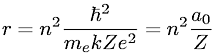Equations > Chemistry > Atomic Structure > Radii of stable orbits in the Bohr model

### Radii of stable orbits in the Bohr modelLatex Code:

MathML Code:

 $r={n}^{2}\frac\hslash {}^{2}{m}_{e}{\mathrm{kZe}}^{2}={n}^{2}\frac{a}_{0}Z$

MathType 5.0: